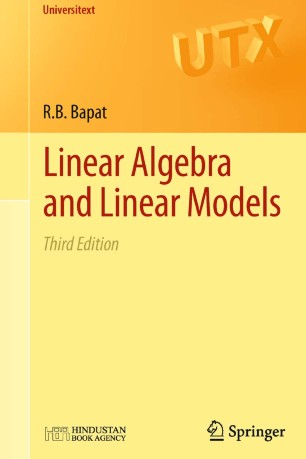# Linear Algebra and Linear Models

• R.B. BapatTextbook

Part of the Universitext book series (UTX)

1. Front Matter
Pages I-VIII
2. R. B. Bapat
Pages 1-8
3. R. B. Bapat
Pages 9-19
4. R. B. Bapat
Pages 21-29
5. R. B. Bapat
Pages 31-36
6. R. B. Bapat
Pages 37-50
7. R. B. Bapat
Pages 51-60
8. R. B. Bapat
Pages 61-77
9. R. B. Bapat
Pages 79-98
10. R. B. Bapat
Pages 99-114
11. R. B. Bapat
Pages 115-128
12. R. B. Bapat
Pages 129-134
13. R. B. Bapat
Pages 135-156
14. R. B. Bapat
Pages 157-159
15. Back Matter
Pages 161-167

### Introduction

Linear Algebra and Linear Models comprises a concise and rigorous introduction to linear algebra required for statistics followed by the basic aspects of the theory of linear estimation and hypothesis testing. The emphasis is on the approach using generalized inverses. Topics such as the multivariate normal distribution and distribution of quadratic forms are included.

For this third edition, the material has been reorganised to develop the linear algebra in the first six chapters, to serve as a first course on linear algebra that is especially suitable for students of statistics or for those looking for a matrix theoretic approach to the subject. Other key features include:

• coverage of topics such as rank additivity, inequalities for eigenvalues and singular values;

• a new chapter on linear mixed models;

• over seventy additional problems on rank: the matrix rank is an important and rich topic with connections to many aspects of linear algebra such as generalized inverses, idempotent matrices and partitioned matrices.

This text is aimed primarily at advanced undergraduate and first-year graduate students taking courses in linear algebra, linear models, multivariate analysis and design of experiments. A wealth of exercises, complete with hints and solutions, help to consolidate understanding. Researchers in mathematics and statistics will also find the book a useful source of results and problems.

### Keywords

linear algebra linear model mixed effects model normal equations variance components variance-covariance matrix

#### Authors and affiliations

• R.B. Bapat
• 1
1. 1.Indian Statistical InstituteNew DelhiIndia